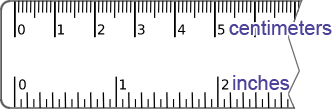# cm to inches

 From millimeter (mm) centimeter (cm) meter (m) kilometer (km) inch (in) foot (ft) yard (yd) mile (mi) To millimeter (mm) centimeter (cm) meter (m) kilometer (km) inch (in) foot (ft) yard (yd) mile (mi) cm = ? in

This converter makes instant conversions from centimeters (cm) to inches (in). It also provides the conversion steps. To use the converter, please provide a value in the input field. The converter will begin making conversions as the values are being typed in.A centimeters (cm) is a unit of length in the International System of Units (SI). In SI, units of measurement are comprised of a base unit and a prefix that describes the magnitude of the unit. The base unit of the centimeters is the meter, and the prefix "centi-" indicates the magnitude 10-2. In other words, a centimeter ismeters.

An inch (in) is a unit of length in the United States (US) customary systems of measurement as well as in the British imperial system. Unlike SI, the relationship between units of measurement within the US customary system is not as clear; it is necessary to know the specific conversions between the units of measurement even within the same system. For example, there are 12 inches in 1 foot and 3 feet in 1 yard, so there are 36 inches in a yard.

### How many inches in a centimeter?

The basis of many units of measurement have changed over the course of history as well as location. During the 1950s and 1960s, the definition of the inch was re-defined to be exactly 25.4 mm or 2.54 cm based on the international yard, which has its basis in the metric system. The adoption of this new definition of the inch took some time, but it is the definition that is still used today. Based on this definition of the inch, there are≈ 0.3937008 inches in a centimeter.

### How to convert centimeters to inches

Based on the definition of 1 inch being exactly 2.54 cm, the centimeter to inch conversion formula is:

inch =
 cm 2.54

Thus, to convert from centimeters to inches, divide a value in centimeters by 2.54. For example, convert 50 centimeters to inches:

50 cm =
 50 2.54
in = 19.685 in